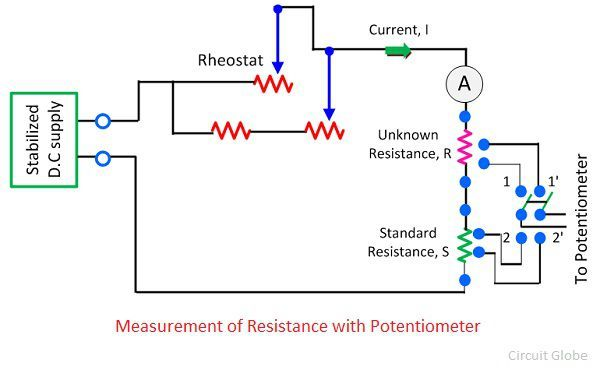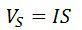# Measurement of Resistance using Potentiometer

The DC potentiometer method of measurement of resistance is used for measuring the unknown resistance of low value. This can be done by comparing the unknown resistance with the standard resistance. The voltage drop across the known and unknown resistance is measured and by comparison the value of known resistance is determined.

Let understand this with the help of the circuit diagram. The R is the unknown resistance whose value is needed to be measured. The S is the standard resistance from which the value of unknown resistance is compared. The rheostat is used for controlling the magnitude of current into the circuit.The double pole double throw switch is used in the circuit. The switch, when moves to position 1, 1  the unknown resistance connects to the circuit, and when it moves to position 2, 2 the standard resistance connects to the circuit.

Consider that when the switch is in position 1,1 the voltage drop across the unknown resistance is Vrand when it is in 2, 2 the voltage drop across the resistance is VsOn equating the equation (1) and (2), we getThe accuracy of unknown resistance depends on the value of standard resistance.

The accuracy of the unknown resistance also depends on the magnitude of the current at the time of the readings. If the magnitude of current remains same, the circuit gives the accurate result. The ammeter is used in the circuit for determining the magnitude of current passing through resistor during the reading.

The magnitude of the current is adjusted in such a way that the voltage drop across the resistance is equal to 1 volt.